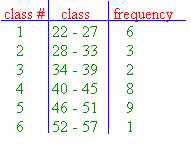### CFA Practice Question

There are 985 practice questions for this topic.

### CFA Practice Question

For the grouped frequency distribution shown below, the class boundaries for class #3 are ______.A. 34 - 39
B. 33.5 - 39.5
C. 34.5 - 38.5

The class boundaries are from the midpoints of the class limits (on a bar graph they are the edges for the bars). For class #3, halfway between 33 and 34 is 33.5 and halfway between 39 and 40 is 39.5. So, the class boundaries are 33.5 - 39.5.

User Comment
yly13 Apparently this is a set of discrete data, so wouldn't A be right?
AyshaAli did not get it?? what are class boundries?
surob I guess I found the interpretation. Class boundaries - the lower/upper limit -/+0.5
Khadria There is no gap between the upper boundary of one class and the lower boundary of the next class. The lower class boundary is found by subtracting 0.5 units from the lower class limit and the upper class boundary is found by adding 0.5 units to the upper class limit. If A would have been correct, then where does one put data "33.5"?
Sumit14 I guess that would depend on your data set...it is whole numbers, integers, real numbers...
Vikku A question:
If class boundaries of #3 are 33.5-39.5
The class boundaries of #4 would be 39.5-45.5 & so on
Now the question is: which class an observation of 39.5 would belong to?
Any suggestions?
mynotes Apparently from the element values in each class, we have positive integers elements. Class boundaries/values explanation is given already and good enough to understand, in the answer area.

Class boundaries are not representing the element values, they are only the containers, that contain elements in them.
2014 Class boundries: Minus .5 from lower Limit, add .5 to each upper limit
Shaan23 2014...not its not .5 from each limit.

it's the midpoint between two class limits. If limits were [2,4] and [8,10] the class bounderies here would be between 4 and 8(midpoint)...which would equal 6